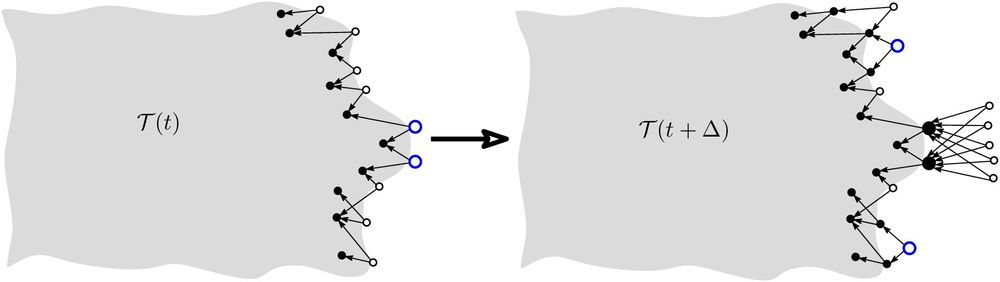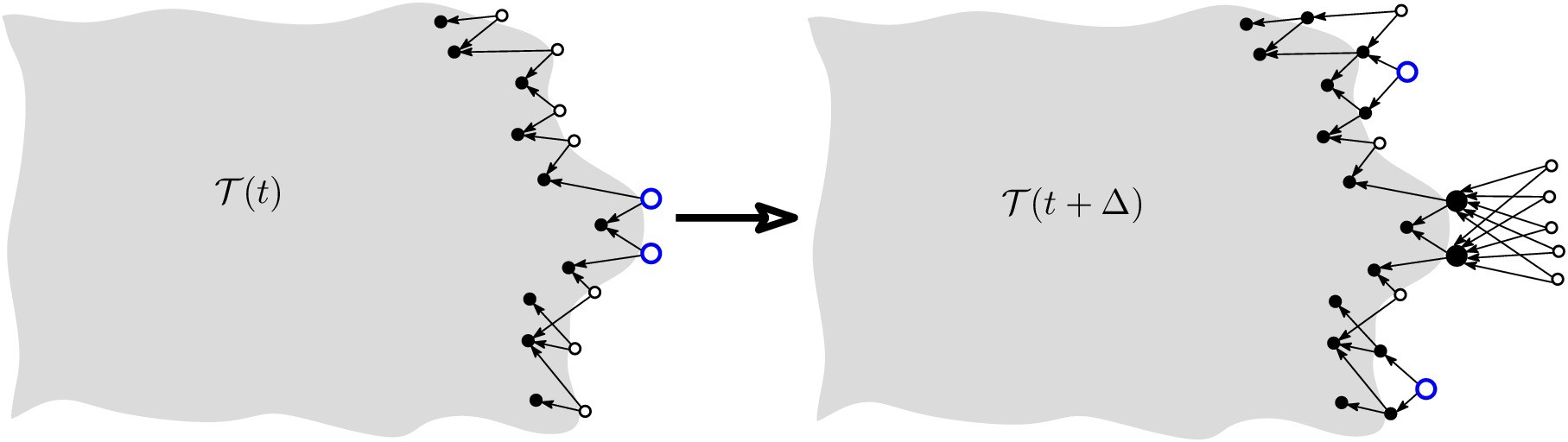Since the early days of IOTA, some questions were raised about the
fact that the default tip-selection algorithm is not enforced— that is, there
is no way to check if a given node really used the recommended MCMC method for selecting tips, or if it tries to tweak it in some way, to obtain advantages for itself. Indeed, as discussed in the whitepaper, for a good functioning of the system the tip selection must be sufficiently random— when a node chooses where to attach its transaction, this choice should be among many tips, with comparable probabilities.

To see why this randomness is important, assume that the nodes always try to choose the best (according to some criterion) two tips. Since there are many nodes around and the transactions’ flow is big, there will be “competition” among those who chose the given two tips that were considered as “best”. Between these competitors, only a few will get lucky (their tips will be considered “the best”), and others will fall into oblivion.

Therefore, it is indeed of concern that the selfish nodes would choose some kind of “greedy” strategy, which would ultimately lead to the situation described above, and, therefore, the system’s failure. How can we investigate this question, to see if this will happen or not? Let us look at some theories first.

In a non-cooperative game between two or more players, a Nash Equilibrium is achieved when — given the strategies of the other players — each of the players has nothing to gain by deviating from his own current strategy. In certain cases, such a Nash Equilibrium can occur where some or all the players are left worse off in comparison to the outcome of a cooperative game, such as the well-known Prisoner’s Dilemma game. In other cases, a Nash Equilibrium can occur in a Pareto-optimal outcome where no other possible outcome would make any player better off without making at least one player worse off.

Because IOTA is a decentralized, distributed and permissionless network of nodes, it must be assumed that some proportion of the nodes in this system will be playing a non-cooperative game, choosing only to maximize their own self-interest. It is therefore important to understand where an equilibrium might occur in this particular game given the presence of selfish (or “greedy”) nodes. Would it devolve into a tragedy of the commons? Or would the Nash Equilibrium occur at a Pareto-efficient outcome? Would a Nash Equilibrium exist at all in this situation?

Almost 70 years ago John Nash proved the existence of equilibria for the case of a finite number of players each having finite sets of possible choices, in a situation when the outcome is determined (i.e. nonrandom) by the players’ choices. This last condition, however, does not hold for the Tangle, since, at the time the transaction is issued, it is not known when (and whether) it will be confirmed. So, in the paper Equilibria in the tangle, we present rigorous proof of the existence of a Nash Equilibrium in the non-cooperative game where some fraction of the nodes choose a greedy tip selection strategy to minimize their cost (for example, the time it takes for their own transactions to be approved).

We also proved that given the number of nodes in the network is large, all Nash equilibria are “almost symmetric”, in the sense that the costs of all nodes are roughly the same, and this, in its turn, permits us to assume that all nodes can adopt the same strategy. We need the latter for the simulations since trying to simulate many nodes with a plethora of different strategies would be unfeasible.

All the above theory, however, does not answer the question we posed before: will the selfish nodes “destroy” the network? To answer this question we need to see what the Nash equilibria look like, but, due to the overall complexity of the system, it seems to be too difficult to obtain a purely analytic solution, even in a (relatively) simple situation when a selfish node chooses (with some probability) the above “greedy” strategy of approving two “best” tips. However, it is still possible to understand why that “naive” greedy tip selection strategy would not be a Nash Equilibrium in the noncooperative game played between greedy nodes.Why the “greedy” tip selection strategy will not work (the two “best” tips are shown as larger blue circles). Many selfish nodes attach their transactions to the two “best” tips believing that by choosing these tips their transactions will be more likely to be chosen by subsequent transactions. As a result, the “neighborhood” of these two tips becomes “overcrowded”: there is so much competition between the transactions issued by the selfish nodes, that the chances they are selected for approval by subsequent transactions actually decrease and they all lose.

Simulations were performed to validate the above intuition, and they showed that it was correct indeed (in a subsequent blog post we will describe these simulations in a much more detailed way). Moreover, in the Nash equilibrium, the system was nearly as efficient as in the “fully cooperative” regime (no selfish nodes) which could suggest a Pareto-optimal Nash Equilibrium. As a concluding remark, we observe that, given that the selfish nodes still have some extra costs (for example, they need to calculate the exit distribution of some Markov chain on a very large state space, which can be computationally expensive), they will have little or no incentive to bother following any fancy tip selection strategies instead of the default one.

Part 2 of this blog post series.